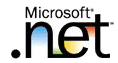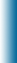Welcome to Mike95.com
 Home Features C++ Library Source Code: COM / ASP Tutorials: Sample Work Internet Based Visual C++ / MFC Java Personal
C++ Templates
Using a class template

Using a class template is easy. Create the required classes by plugging in the actual type for the type parameters. This process is commonly known as "Instantiating a class". Here is a sample driver class that uses the Stack class template.

```#include <iostream>
#include "stack.h"
using namespace std ;
void main()
{
typedef Stack<float> FloatStack ;
typedef Stack<int> IntStack ;

FloatStack fs(5) ;
float f = 1.1 ;
cout << "Pushing elements onto fs" << endl ;
while (fs.push(f))
{
cout << f << ' ' ;
f += 1.1 ;
}
cout << endl << "Stack Full." << endl
<< endl << "Popping elements from fs" << endl ;
while (fs.pop(f))
cout << f << ' ' ;
cout << endl << "Stack Empty" << endl ;
cout << endl ;

IntStack is ;
int i = 1.1 ;
cout << "Pushing elements onto is" << endl ;
while (is.push(i))
{
cout << i << ' ' ;
i += 1 ;
}
cout << endl << "Stack Full" << endl
<< endl << "Popping elements from is" << endl ;
while (is.pop(i))
cout << i << ' ' ;
cout << endl << "Stack Empty" << endl ;
}
```

### Program Output

```Pushing elements onto fs
1.1 2.2 3.3 4.4 5.5
Stack Full.

Popping elements from fs
5.5 4.4 3.3 2.2 1.1
Stack Empty

Pushing elements onto is
1 2 3 4 5 6 7 8 9 10
Stack Full

Popping elements from is
10 9 8 7 6 5 4 3 2 1
Stack Empty
```

In the above example we defined a class template Stack. In the driver program we instantiated a Stack of float (FloatStack) and a Stack of int(IntStack). Once the template classes are instantiated you can instantiate objects of that type (for example, fs and is.)

A good programming practice is using typedef while instantiating template classes. Then throughout the program, one can use the typedef name. There are two advantages:

• typedef's are very useful when "templates of templates" come into usage. For example, when instantiating an STL vector of int's, you could use:
```typedef vector<int, allocator<int> > INTVECTOR;
```
• If the template definition changes, simply change the typedef definition. For example, currently the definition of template class vector requires a second parameter.
```typedef vector<int, allocator<int> > INTVECTOR; INTVECTOR vi1;
```
In a future version, the second parameter may not be required, for example,
```typedef vector<int> INTVECTOR; INTVECTOR vi1;
```
Imagine how many changes would be required if there was no typedef!
 (c)2023 Mike95.com / Site Disclaimer